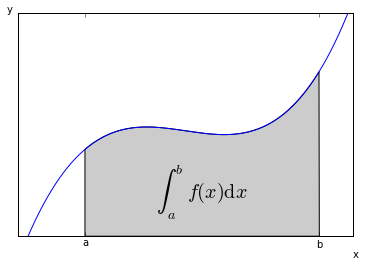# Typesetting Math Using MathJax

The Markdown parser included in IPython is MathJax-aware. This means that you can freely mix in mathematical expressions using the MathJax subset of Tex and LaTeX. Some examples from the MathJax site are reproduced below, as well as the Markdown+TeX source.

Other Reference:

# Inline Typesetting (Mixing Markdown and TeX)

While display equations look good for a page of samples, the ability to mix math and formatted text in a paragraph is also important.

This expression $\sqrt{3x-1}+(1+x)^2$ is an example of a TeX inline equation in a Markdown-formatted sentence.

## Source

This expression $\sqrt{3x-1}+(1+x)^2$ is an example of a TeX inline equation in a **[Markdown-formatted](http://daringfireball.net/projects/markdown/)** sentence.

# Other Syntax

You will notice in other places on the web that $$ are needed explicitly to begin and end MathJax typesetting. This is not required if you will be using TeX environments, but the IPython notebook will accept this syntax on legacy notebooks. ### Source $$ \begin{array}{c} y_1 \\\ y_2 \mathtt{t}_i \\\ z_{3,4} \end{array} $$ $$
\begin{array}{c}
y_1 \cr
y_2 \mathtt{t}_i \cr
y_{3}
\end{array}
$$ $$\begin{eqnarray}
x' &=& &x \sin\phi &+& z \cos\phi \\
z' &=& - &x \cos\phi &+& z \sin\phi \\
\end{eqnarray}$$ $$
x=4


### Display

$\begin{array}{c} y_1 \\\ y_2 \mathtt{t}_i \\\ z_{3,4} \end{array}$

$\begin{array}{c} y_1 \cr y_2 \mathtt{t}_i \cr y_{3} \end{array}$

$\begin{eqnarray} x' &=& &x \sin\phi &+& z \cos\phi \\ z' &=& - &x \cos\phi &+& z \sin\phi \\ \end{eqnarray}$

$x=4$

# Examples

## The Lorenz Equations

### Source

\begin{equation*}
\dot{x} = \sigma(y-x)
\end{equation*}
\begin{equation*}
\dot{y} = \rho x - y - xz
\end{equation*}
\begin{equation*}
\dot{z} = -\beta z + xy
\end{equation*}

## Euler's Formula

### Source

\begin{equation*} e ^{i \theta} = \cos{\theta} + i \sin{\theta} \end{equation*} \begin{equation*} e ^{i \pi} + 1 = 0 \end{equation*}

## The Cauchy-Schwarz Inequality

### Source

\begin{equation*} \left( \sum_{k=1}^n a_k b_k \right)^2 \leq \left( \sum_{k=1}^n a_k^2 \right) \left( \sum_{k=1}^n b_k^2 \right) \end{equation*}

## Fourier Transform

### Source

\begin{equation*} X(\omega) = \int_{-\infty}^{\infty} x(t) e ^{-j \omega t} \mathrm{d}t = \int_{-\infty}^{\infty} x(t) e ^{-j 2 \pi f t} \mathrm{d}t \end{equation*}

\begin{equation*} x(t) = \frac{1}{2 \pi} \int_{-\infty}^{\infty} X(\omega) e ^{j \omega t} \mathrm{d}\omega = \int_{-\infty}^{\infty} X(f) e ^{j 2 \pi f t} \mathrm{d}f \end{equation*}

\begin{equation*} x(t) \Leftrightarrow X(w) \end{equation*}

## Fourier Series

A function is said to have period $P$ if $f(x + P) = f(x) \hspace{8pt}$ for all $x$. For notational simplicity, we shall restrict our discussion to functions of period $2 \pi$. There is no loss of generality in doing so, since we can always use a simple change of scale $x = \left( \frac{P}{2 \pi} \right) t \hspace{5pt}$ to convert a function of period $P$ into one of period $2 \pi$. If the function $f$ has period $2 \pi$, then its Fourier series of $f\left(x\right) \hspace{5pt}$ is

with Fourier coefﬁcients $c_0$, $a_n$, and $b_n$ deﬁned by the integrals

It is more common nowadays to express Fourier series in an algebraically simpler form involving complex exponentials. Following Euler Formula, we use the fact that the complex exponential $e^{i \theta}$ satisfies $e ^{i \theta} = \cos{\theta} + i \sin{\theta} \hspace{5pt}$. Then the Fourier Series can be rewriten

where

## Dirichlet Kernel

### Source

\begin{equation*} D_n(x) = \sum_{k = - n}^{n} e^{i k x} = 1 + 2 \sum_{k = 1}^{n} \cos(k x) = \frac{\sin((n + \frac{1}{2}) x)}{\sin(x / 2)} \end{equation*}

### Source

\begin{equation*} x = \frac{-b \pm \sqrt{b^{2} -4ac}}{2a} \end{equation*}

## Matrixes

### Display







$A =$

,

B =

$\left(A|B\right)= \left[\right].$

## A Cross Product Formula

### Source

\begin{equation*} \mathbf{V}_1 \times \mathbf{V}_2 = \begin{vmatrix} \mathbf{i} & \mathbf{j} & \mathbf{k} \\ \frac{\partial X}{\partial u} & \frac{\partial Y}{\partial u} & 0 \\ \frac{\partial X}{\partial v} & \frac{\partial Y}{\partial v} & 0 \end{vmatrix} \end{equation*} ### Display

## The probability of getting (k) heads when flipping (n) coins is

### Source

\begin{equation*} P(E) = {n \choose k} p^k (1-p)^{ n-k} \end{equation*} ### Display

## An Identity of Ramanujan

### Source

\begin{equation*} \frac{1}{\Bigl(\sqrt{\phi \sqrt{5}}-\phi\Bigr) e^{\frac25 \pi}} = 1+\frac{e^{-2\pi}} {1+\frac{e^{-4\pi}} {1+\frac{e^{-6\pi}} {1+\frac{e^{-8\pi}} {1+\ldots} } } } \end{equation*} ### Display

## A Rogers-Ramanujan Identity

### Source

\begin{equation*} 1 + \frac{q^2}{(1-q)}+\frac{q^6}{(1-q)(1-q^2)}+\cdots = \prod_{j=0}^{\infty}\frac{1}{(1-q^{5j+2})(1-q^{5j+3})}, \quad\quad \text{for $|q|<1$}. \end{equation*} ### Display

## Maxwell's Equations

### Source

\begin{aligned} \nabla \times \vec{\mathbf{B}} -\, \frac1c\, \frac{\partial\vec{\mathbf{E}}}{\partial t} & = \frac{4\pi}{c}\vec{\mathbf{j}} \\ \nabla \cdot \vec{\mathbf{E}} & = 4 \pi \rho \\ \nabla \times \vec{\mathbf{E}}\, +\, \frac1c\, \frac{\partial\vec{\mathbf{B}}}{\partial t} & = \vec{\mathbf{0}} \\ \nabla \cdot \vec{\mathbf{B}} & = 0 \end{aligned} ### Display

$-, 1c, & = \ & = 4 \ , +, 1c, & = \ & = 0 <>$

## Schrödinger Equation

Time-dependent Schrödinger equation (general)

Time-dependent Schrödinger equation (single non-relativistic particle)

Time-independent Schrödinger equation (general)

Time-independent Schrödinger equation (single non-relativistic particle)

### Source

\begin{equation*} i \hbar \frac{\partial{}}{\partial{t}} \Psi = \hat{H} \Psi \end{equation*}

\begin{equation*} i \hbar \frac{\partial{}}{\partial{t}} \Psi(r,t) = \left[\frac{-\hbar^2}{2 m} \nabla^2 +V(r,t)\right] \Psi(r,t) \end{equation*}

\begin{equation*} E \Psi = \hat{H} \Psi \end{equation*}

\begin{equation*} E \Psi(r) = \frac{-\hbar^2}{2 m} \nabla^2 \Psi(r) + V(r) \Psi(r) \end{equation*}

## Imbedding MathJax in Plots

In :
from pylab import *
from matplotlib.patches import Polygon

def func(x):
return (x-3)*(x-5)*(x-7)+85

ax = subplot(111)

a, b = 2, 9 # integral area
x = arange(0, 10, 0.01)
y = func(x)
plot(x, y, linewidth=1)

# make the shaded region
ix = arange(a, b, 0.01)
iy = func(ix)
verts = [(a,0)] + list(zip(ix,iy)) + [(b,0)]
poly = Polygon(verts, facecolor='0.8', edgecolor='k')

# text for the intergal
text(0.5 * (a + b), 30, r"$\int_a^b f(x)\mathrm{d}x$", horizontalalignment='center', fontsize=20)

# axis text
axis([0,10, 0, 180])
figtext(0.9, 0.05, 'x')
figtext(0.1, 0.9, 'y')
ax.set_xticks((a,b))
ax.set_xticklabels(('a','b'))
ax.set_yticks([])
show()Adapted From: Brian E. Granger's Git repository